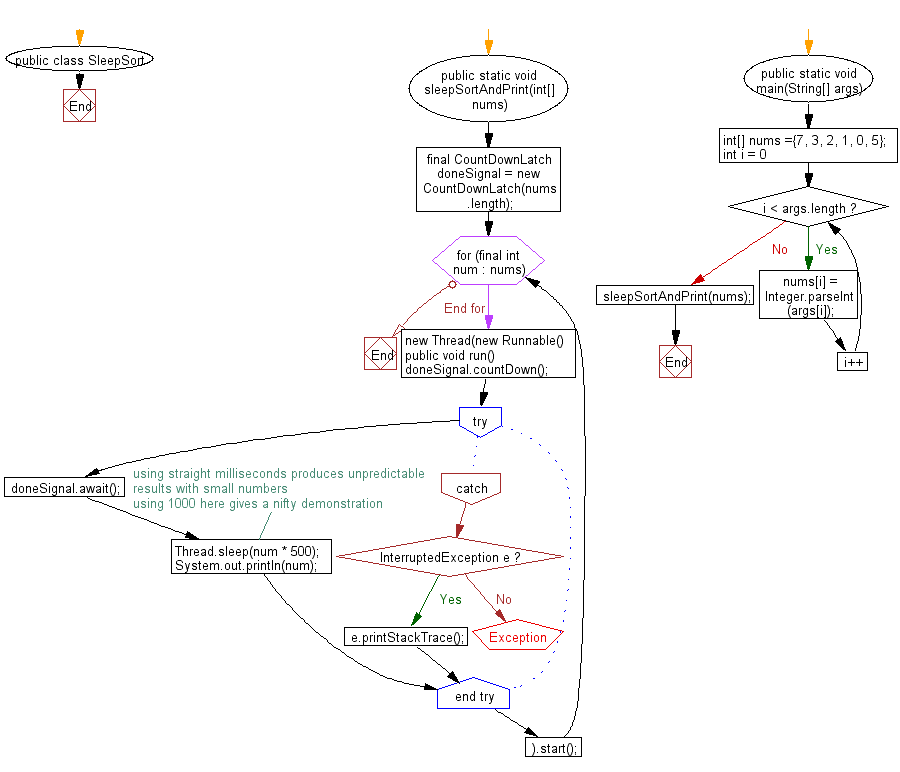﻿ Java exercises: Sleep Sort Algorithm - w3resource# Java Exercises: Sleep Sort Algorithm

## Java Sorting Algorithm: Exercise-17 with Solution

Write a Java program to sort an array of given non-negative integers using Sleep Sort Algorithm.

In general, sleep sort works by starting a separate task for each item to be sorted, where each task sleeps for an interval corresponding to the item's sort key, then emits the item. Items are then collected sequentially in time.

Sample Solution:

Java Code:

``````import java.util.concurrent.CountDownLatch;

public class SleepSort {
public static void sleepSortAndPrint(int[] nums) {
final CountDownLatch doneSignal = new CountDownLatch(nums.length);
for (final int num : nums) {
public void run() {
doneSignal.countDown();
try {
doneSignal.await();

//using straight milliseconds produces unpredictable
//results with small numbers
//using 1000 here gives a nifty demonstration
System.out.println(num);
} catch (InterruptedException e) {
e.printStackTrace();
}
}
}).start();
}
}
public static void main(String[] args) {
int[] nums ={7, 3, 2, 1, 0, 5};
for (int i = 0; i < args.length; i++)
nums[i] = Integer.parseInt(args[i]);
sleepSortAndPrint(nums);
}
}
```
```

Sample Output:

```0
1
2
3
5
7
```

Flowchart:Java Code Editor: